• 多元 logistic 回归 1. 下面是子宫内膜癌的病例对照研究数据暴露因素是雌激素 组 使用过雌激素 未使用过雌激素 病例组 55 a 128 b 对照组 19 c 164 d 问题使用过雌激素是否是子宫内膜癌的危险因素危险强度为多少 2. ...
• 多元Logistic_回归分析多元Logistic_
• 如何用spss17.0进行二元和多元logistic回归分析
• 分析——回归——有序输出——勾选平行线检验(作用：检验是否需要进行有序多分类)、预测类别、预测类别概率结果解读：因变量学历共有5个取值，因此一共要切割四次，做4个二元回归模型模型的P<0.05，说明至少有一...
分析——回归——有序输出——勾选平行线检验(作用：检验是否需要进行有序多分类)、预测类别、预测类别概率结果解读：因变量学历共有5个取值，因此一共要切割四次，做4个二元回归模型模型的P<0.05，说明至少有一个自变量对因变量有显著作用拟合优度检验的P>0.05，说明模型还可以接下俩看平行性检验是否通过(P>0.05通过)。如果未通过，就不能建立有序多分类模型原假设：在因变量的不同切割点建立的二元逻辑回归模型中，自变量对因变量的影响程度是一样的。这里P=0.097>0.05通过平行性检验，可以建立有序多分类模型最后看参数显著性一共切割了4次，第一次学历=1的取值与学历=2、3、4、5的取值建立二元回归；第二次学历=1、2与学历=3、4、5的取值建立二元回归……根据检验结果可以看到，性别、是否已婚对因变量影响作用不显著。可以把这两个变量去掉再建立一次逻辑回归删去影响作用不显著的变量，再次建模结果：模型的显著性P<0.05，说明至少有一个自变量对因变量有显著影响拟合优度检验的显著性P>0.05，说明模型拟合的结果可以接受平行性检验P=0.095>0.05，通过平行线检验，可以建立有序多分类模型写模型 一共切割了4次，所以要写4个ln(P1/(1-P1))=-2.494-0.032*age+0.003*income          p1表示学历取1的概率解读：年龄每增加一个单位，优势比变为e的-0.032次方倍(B<0,OR<1)，即年龄越大，学历取1的概率就会变小(年龄越大学历越高)收入每增加一个单位，优势比变为e的0.003次方倍(B>0,OR>1)ln(P2/(1-P2))=-1.110-0.032*age+0.003*income          p2表示学历取1、2的概率ln(P3/(1-P3))=-0.192-0.032*age+0.003*income          p3表示学历取1、2、3的概率ln(P4/(1-P4))=1.649-0.032*age+0.003*income           p4表示学历取1、2、3、4的概率瓦尔德是影响大小e的估算次幂=OR优势比展开全文• 概念多元 logistics 回归(multinomial logistics regression)又称多分类 logistics 回归。医学研究、社会科学领域中， 存在因变量是多项的情况， 其中又分为无序(口味：苦、 甜、 酸、 辣；科目：数学、 自然、 ...


文章作者 | 赵利娟  文章来源 | SPSS学堂01. 概念多元 logistics 回归(multinomial logistics regression)又称多分类 logistics 回归。医学研究、社会科学领域中， 存在因变量是多项的情况， 其中又分为无序(口味：苦、 甜、 酸、 辣；科目：数学、 自然、 语文、 英语) 和有序(辣度：微辣、 中辣、 重辣) 两类。对于这类数据需要用多元 logistics 回归。多元 logistics 回归实际就是多个二元 logistics 回归模型描述各类与参考分类相比各因素的作用。如， 对于一个三分类的因变量(口味：酸、甜、辣)，可建立两个二元logistics回归模型，分别描述酸味与甜味相比及辣味与酸味相比，各口味的作用。但在估计这些模型参数时，所有对象是一起估计的，其他参数的意义及模型的筛选等与二元logistics类似。02.条件因变量：三个及以上分类变量自变量：分类或连续变量协变量：分类变量03.案例及操作【例】为了研究饮食口味偏好的影响因素，分析年龄、婚姻情况、生活态度在饮食口味类型偏好(1=酸、2=甜、3=辣) 中的作用，共挑选被试30人，结果见下表，试进行多元logistics回归。说明：本案例数据纯属编造，结论不具有参考性和科学性， 仅供操作训练使用。⑴ 建立数据文件口味偏好,sav， 见下图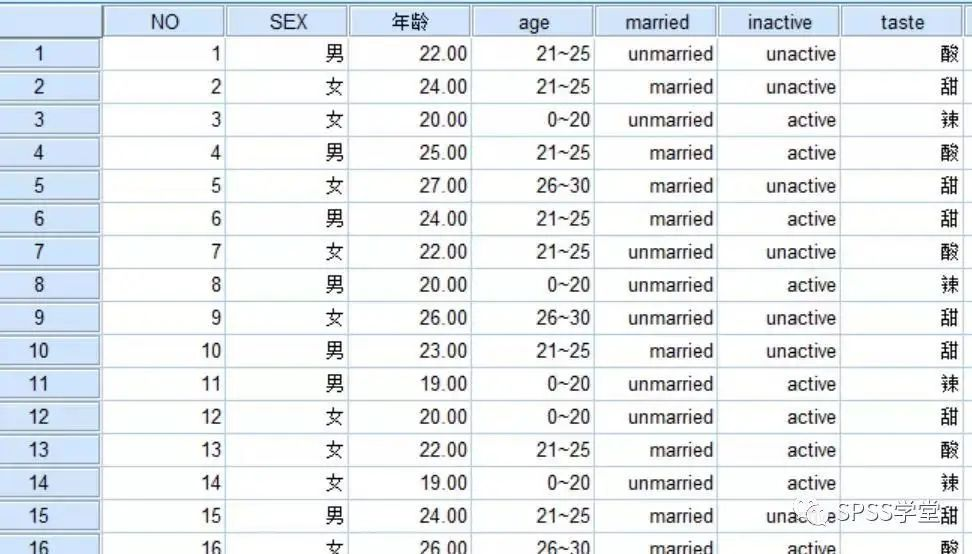每个被试有一个口味偏好因变量taste和3个自变量age、married、inactive。⑵对口味偏好 taste 加权单击【数据】→【加权个案】，打开加权个案对话框， 加权口味偏好，见下图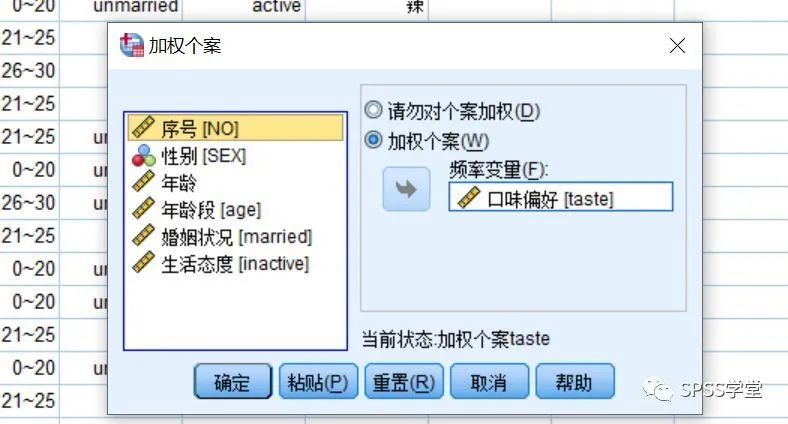(3)选择【分析】→【回归】→【多项logistics】，打开多项 logistics回归主对话框，见图。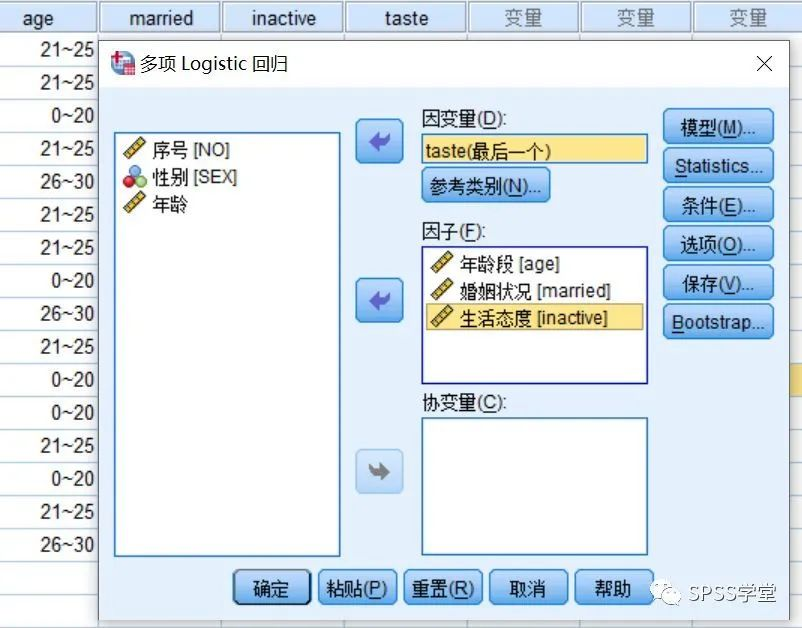 【因变量】：分类变量，本例选择“taste” 【因子】：可选择多个变量作为因子，本例选择“age”、 “married”、“inactive” 【协变量】 ：可选择多个变量作为协变量，本例未选择(4)单击【参考类别】按钮，打开参考类别对话框，见图 【参考类别】：可选择【第一类别】、【最后类别】或【定制】，本例选择【最后类别】 【类别顺序】：可选择【升序】或【降序】(5)单击【模型】按钮，打开模型对话框，见下图：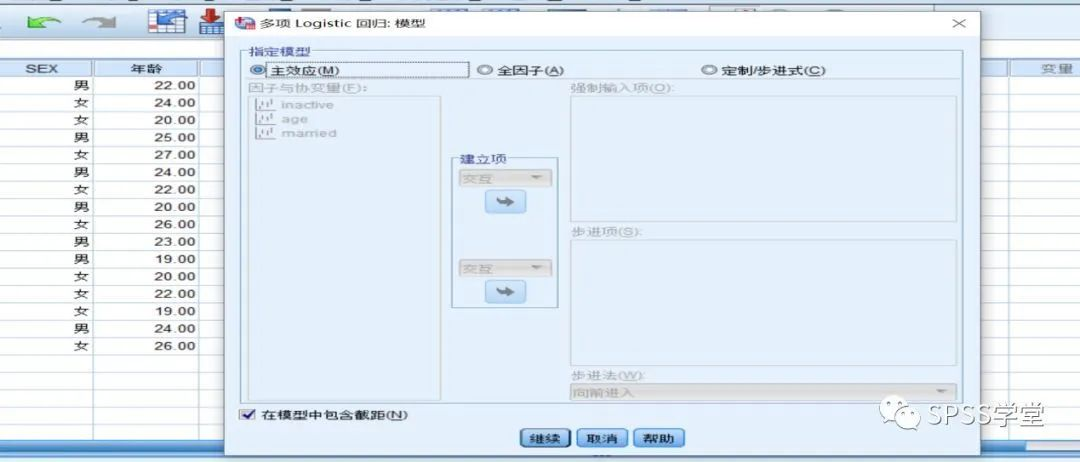本例主要考察自变量age、married、inactive的主效应， 暂不考察它们之间的交互作用，然后点击【继续】；(6)单击【statistics】按钮，打开统计对话框，见图：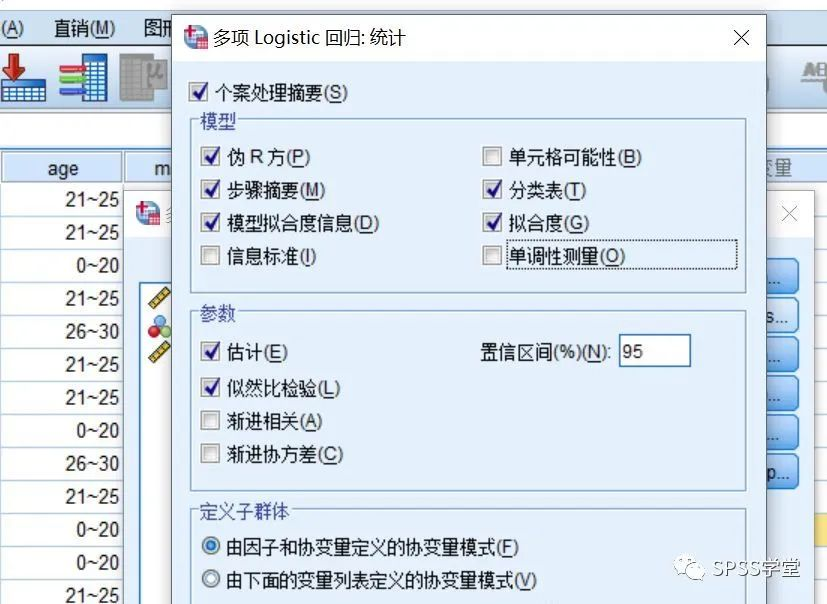设置模型的统计量。主要【伪R方】【模型拟合信息】【分类表】【拟合优度】这几项必选，其他可以默认不勾选。这些参数主要用于说明建模的质量。(7)单击【条件】按钮，打开收敛性准则对话框，见下图，默认选项，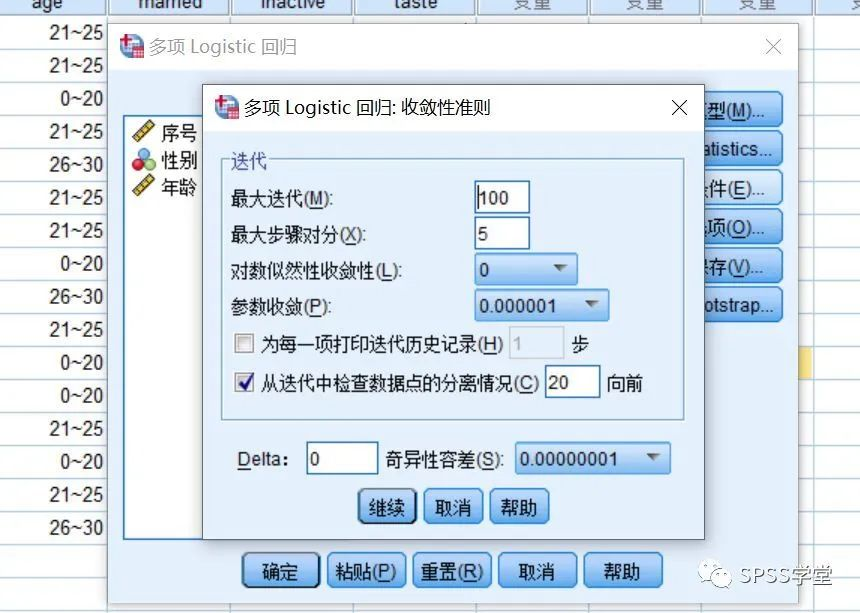(8) 单击【选项】 按钮， 打开选项对话框， 默认选项， 见下图，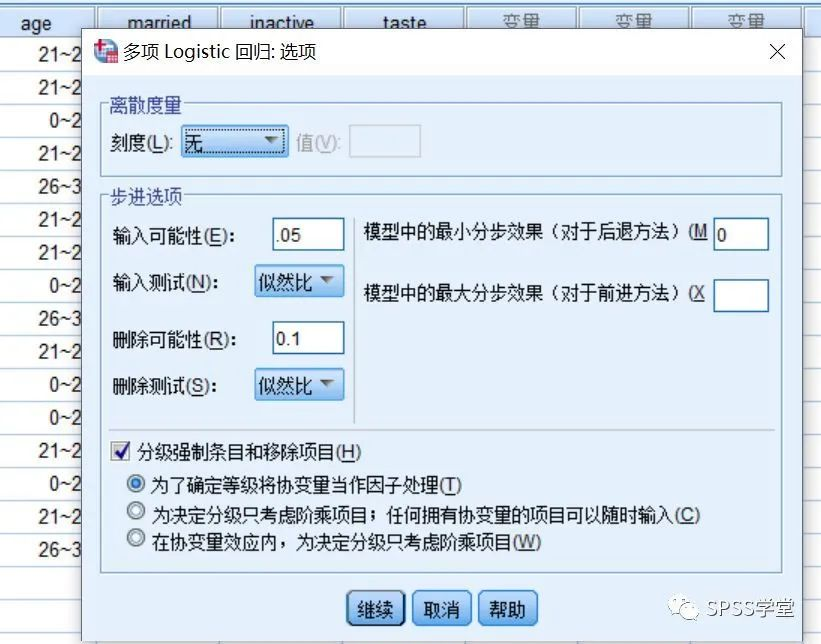(9)单击【保存】按钮，打开保存对话框，勾选【估算响应概率】，估算每个个案三类口味偏好的概率。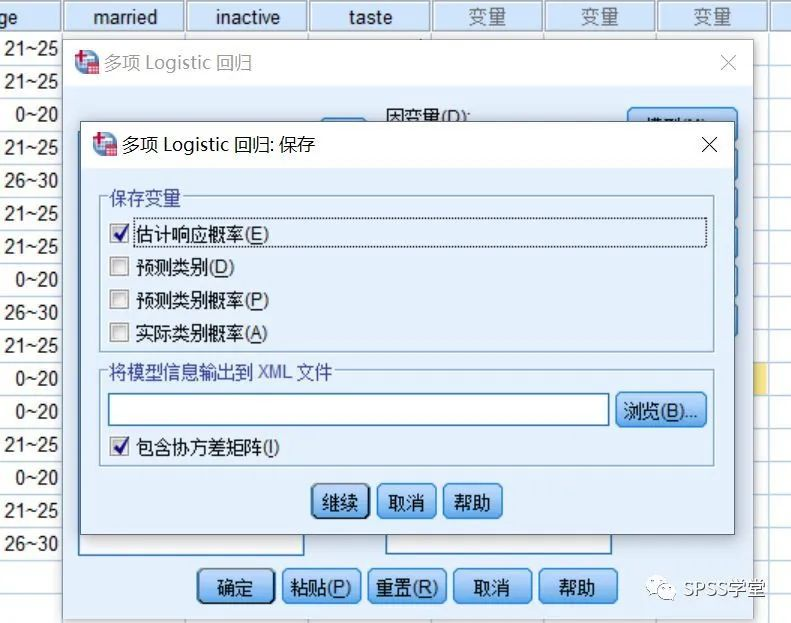(10)单击【确定】 按钮， 得到以下主要结果。04.结果解释结果 1 【个案处理摘要表】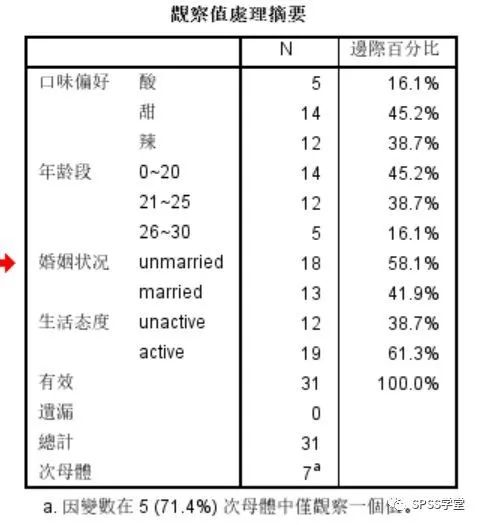列出因变量和自变量的分类水平及对应的个案百分比。建议在此表主要读取变量分类水平的顺序，比如自变量“年龄段”，第一个分类是“0~20 岁”，第二个分类是“21~25”，第三个分类是“26~30”，尤其是看清楚最后一个分类，因为前面参数设置时要求是以最后一个分类最为对比参照组的。结果 2 【模型拟合信息表】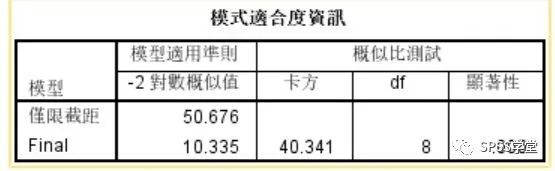读取最后一列，显著性值小于 0.05，说明模型有统计意义，模型通过检验。结果 3 【拟合优度表】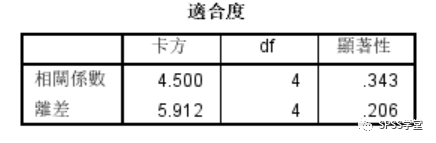原假设模型不能很好地拟合原始数据，最后一列皮尔逊卡方显著性值 0.343，概率较小，原假设不成立，说明模型对原始数据的拟合没有通过检验。结果 4【伪 R 方表】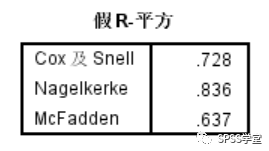依次列出的 3 个伪 R 方值(类似于决定系数)均偏低，最高 0.836，说明模型对原始变量变异的解释程度较好，只有一小部分信息无法解释，拟合程度比较优秀。结果 5【模型似然比检验表】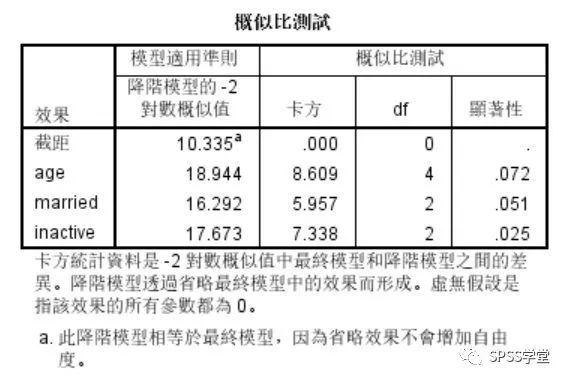最终进入模型的效应包括截距、年龄、婚姻状况、生活态度，而且最后一列显著性值表明，只有生活态度对模型构成有显著贡献。结果 6【参数估计表】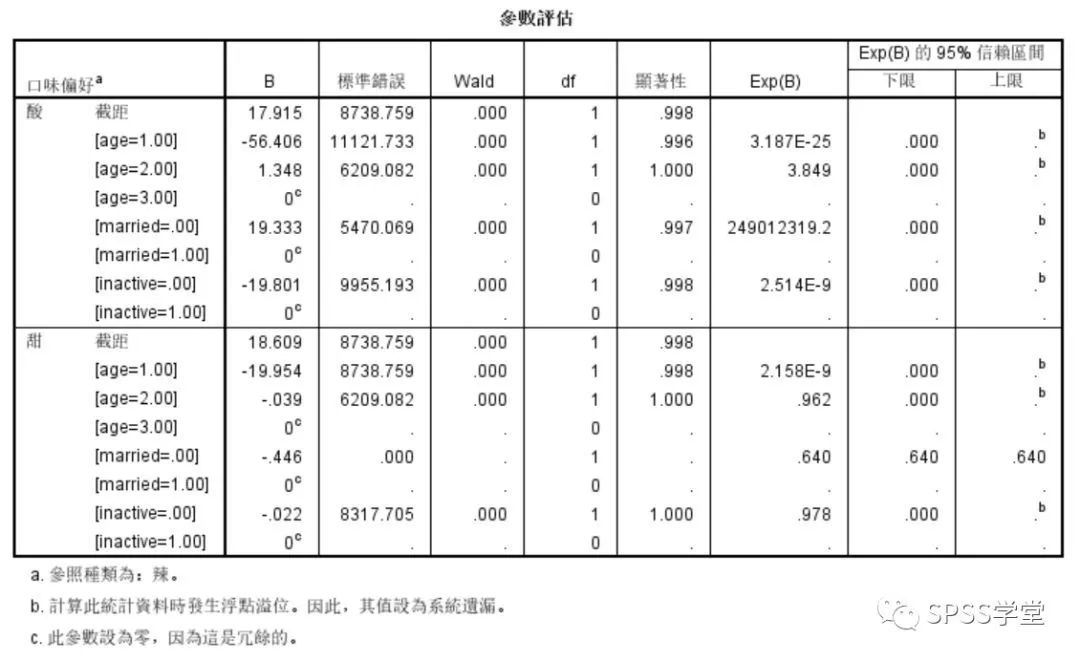列出自变量不同分类水平对口味偏好的影响检验，是多项 logistic 回归非常重要的结果。第二列 B 值，即各自变量不同分类水平在模型中的系数，正负符号表明它们与早餐选择是正比还是反比关系。第六列是瓦尔德检验显著性值，此值小于 0.05 说明对应自变量的系数具有统计意义，对因变量不同分类水平的变化有显著影响。比如，酸和辣相比，21~25岁的年轻人更偏向于选择在酸，这种可能性是 26~30 岁以上人的 3.8 倍；甜和辣相比，结婚与否对口味偏好没有差别。05.多项 logistic 回归模型经过对该口味偏好调查数据进行多项 logistic 回归分析， 由参数估计表，我们可以得到模型如下：G1=LOG[P(酸)/P(辣)]=17.915-56.406age1+1.348age2+19.333married0-19.801inactive0G2=LOG[P(甜)/P(辣)]=18.609-19.954age1-0.039age2-0.446married0-.022inactive0G3=0 (对照组)根据这个模型，我们首先计算某个受访者 G1、G2、G3 的值，然后带入如下公式，最终可得到三个早餐相应的概率。P1=exp(G1)/[exp(G1)+exp(G2)+exp(G3)]P2=exp(G2)/[exp(G1)+exp(G2)+exp(G3)]P3=exp(G3)/[exp(G1)+exp(G2)+exp(G3)]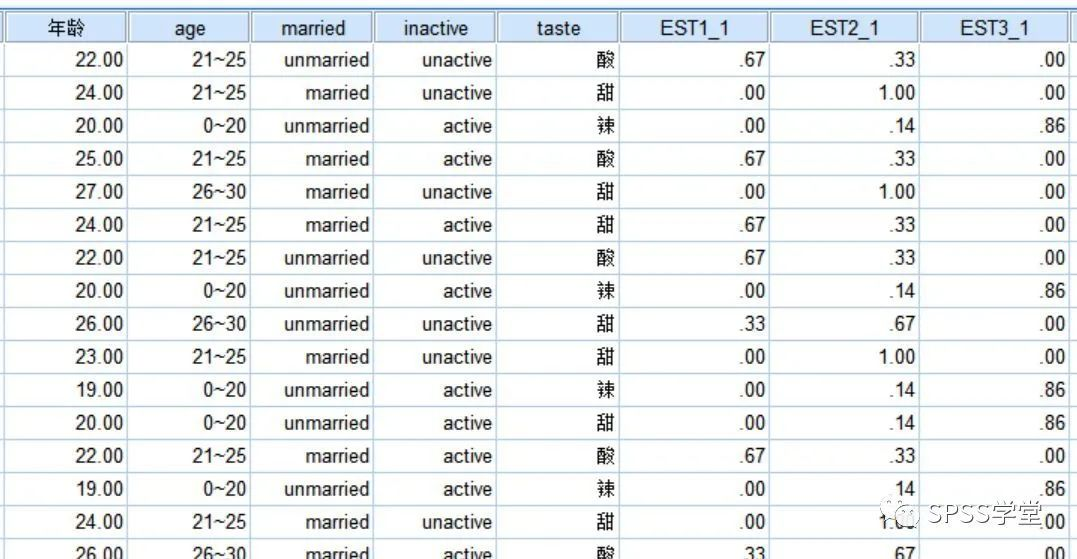原始数据最右侧新增3个变量，依次为EST1_1、EST2_1、EST3_1，分别对应因变量“口味偏好”的三个分类水平(酸、 甜、 辣)的响应概率。比如第一个个案，他选择酸的概率为0.67，在三种选择中数值最大，因此，模型会判定他选择酸，这和原始记录的真值一致，说明模型判断准确。结果 7【分类】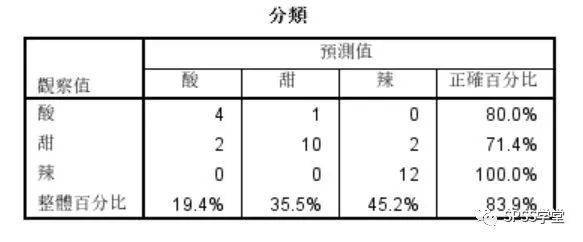模型在预测辣味偏好倾向上准确率最高，达到100%，其他两个口味偏好的预测略低，模型总体预测准确率为83.9%，表现比较好。前面伪 R 方数据显示，模型对总体变异的解释能力尚可，这和总体预测准确率结论也一致。以上就是本节的全部内容，请大家多多练习~

展开全文• 概念多元 logistics 回归(multinomial logistics regression)又称多分类 logistics 回归。医学研究、社会科学领域中， 存在因变量是多项的情况， 其中又分为无序(口味：苦、 甜、 酸、 辣；科目：数学、 自然、 ...


文章作者 | 赵利娟  文章来源 | SPSS学堂01. 概念多元 logistics 回归(multinomial logistics regression)又称多分类 logistics 回归。医学研究、社会科学领域中， 存在因变量是多项的情况， 其中又分为无序(口味：苦、 甜、 酸、 辣；科目：数学、 自然、 语文、 英语) 和有序(辣度：微辣、 中辣、 重辣) 两类。对于这类数据需要用多元 logistics 回归。多元 logistics 回归实际就是多个二元 logistics 回归模型描述各类与参考分类相比各因素的作用。如， 对于一个三分类的因变量(口味：酸、甜、辣)，可建立两个二元logistics回归模型，分别描述酸味与甜味相比及辣味与酸味相比，各口味的作用。但在估计这些模型参数时，所有对象是一起估计的，其他参数的意义及模型的筛选等与二元logistics类似。02.条件因变量：三个及以上分类变量自变量：分类或连续变量协变量：分类变量03.案例及操作【例】为了研究饮食口味偏好的影响因素，分析年龄、婚姻情况、生活态度在饮食口味类型偏好(1=酸、2=甜、3=辣) 中的作用，共挑选被试30人，结果见下表，试进行多元logistics回归。说明：本案例数据纯属编造，结论不具有参考性和科学性， 仅供操作训练使用。⑴ 建立数据文件口味偏好,sav， 见下图每个被试有一个口味偏好因变量taste和3个自变量age、married、inactive。⑵对口味偏好 taste 加权单击【数据】→【加权个案】，打开加权个案对话框， 加权口味偏好，见下图(3)选择【分析】→【回归】→【多项logistics】，打开多项 logistics回归主对话框，见图。 【因变量】：分类变量，本例选择“taste” 【因子】：可选择多个变量作为因子，本例选择“age”、 “married”、“inactive” 【协变量】 ：可选择多个变量作为协变量，本例未选择(4)单击【参考类别】按钮，打开参考类别对话框，见图 【参考类别】：可选择【第一类别】、【最后类别】或【定制】，本例选择【最后类别】 【类别顺序】：可选择【升序】或【降序】(5)单击【模型】按钮，打开模型对话框，见下图：本例主要考察自变量age、married、inactive的主效应， 暂不考察它们之间的交互作用，然后点击【继续】；(6)单击【statistics】按钮，打开统计对话框，见图：设置模型的统计量。主要【伪R方】【模型拟合信息】【分类表】【拟合优度】这几项必选，其他可以默认不勾选。这些参数主要用于说明建模的质量。(7)单击【条件】按钮，打开收敛性准则对话框，见下图，默认选项，(8) 单击【选项】 按钮， 打开选项对话框， 默认选项， 见下图，(9)单击【保存】按钮，打开保存对话框，勾选【估算响应概率】，估算每个个案三类口味偏好的概率。(10)单击【确定】 按钮， 得到以下主要结果。04.结果解释结果 1 【个案处理摘要表】列出因变量和自变量的分类水平及对应的个案百分比。建议在此表主要读取变量分类水平的顺序，比如自变量“年龄段”，第一个分类是“0~20 岁”，第二个分类是“21~25”，第三个分类是“26~30”，尤其是看清楚最后一个分类，因为前面参数设置时要求是以最后一个分类最为对比参照组的。结果 2 【模型拟合信息表】读取最后一列，显著性值小于 0.05，说明模型有统计意义，模型通过检验。结果 3 【拟合优度表】原假设模型不能很好地拟合原始数据，最后一列皮尔逊卡方显著性值 0.343，概率较小，原假设不成立，说明模型对原始数据的拟合没有通过检验。结果 4【伪 R 方表】依次列出的 3 个伪 R 方值(类似于决定系数)均偏低，最高 0.836，说明模型对原始变量变异的解释程度较好，只有一小部分信息无法解释，拟合程度比较优秀。结果 5【模型似然比检验表】最终进入模型的效应包括截距、年龄、婚姻状况、生活态度，而且最后一列显著性值表明，只有生活态度对模型构成有显著贡献。结果 6【参数估计表】列出自变量不同分类水平对口味偏好的影响检验，是多项 logistic 回归非常重要的结果。第二列 B 值，即各自变量不同分类水平在模型中的系数，正负符号表明它们与早餐选择是正比还是反比关系。第六列是瓦尔德检验显著性值，此值小于 0.05 说明对应自变量的系数具有统计意义，对因变量不同分类水平的变化有显著影响。比如，酸和辣相比，21~25岁的年轻人更偏向于选择在酸，这种可能性是 26~30 岁以上人的 3.8 倍；甜和辣相比，结婚与否对口味偏好没有差别。05.多项 logistic 回归模型经过对该口味偏好调查数据进行多项 logistic 回归分析， 由参数估计表，我们可以得到模型如下：G1=LOG[P(酸)/P(辣)]=17.915-56.406age1+1.348age2+19.333married0-19.801inactive0G2=LOG[P(甜)/P(辣)]=18.609-19.954age1-0.039age2-0.446married0-.022inactive0G3=0 (对照组)根据这个模型，我们首先计算某个受访者 G1、G2、G3 的值，然后带入如下公式，最终可得到三个早餐相应的概率。P1=exp(G1)/[exp(G1)+exp(G2)+exp(G3)]P2=exp(G2)/[exp(G1)+exp(G2)+exp(G3)]P3=exp(G3)/[exp(G1)+exp(G2)+exp(G3)]原始数据最右侧新增3个变量，依次为EST1_1、EST2_1、EST3_1，分别对应因变量“口味偏好”的三个分类水平(酸、 甜、 辣)的响应概率。比如第一个个案，他选择酸的概率为0.67，在三种选择中数值最大，因此，模型会判定他选择酸，这和原始记录的真值一致，说明模型判断准确。结果 7【分类】模型在预测辣味偏好倾向上准确率最高，达到100%，其他两个口味偏好的预测略低，模型总体预测准确率为83.9%，表现比较好。前面伪 R 方数据显示，模型对总体变异的解释能力尚可，这和总体预测准确率结论也一致。以上就是本节的全部内容，请大家多多练习~

展开全文• 1. softmax函数 2. 与二元Logistic回归的关系 3. 误差函数 3.1 多元回归的1-of-K表示 3.2 训练样本集的似然函数 3.3 交叉熵误差函数 4. 最大似然估计 代码实现（mnist数据集）
Multi-class Logistic Regression1. softmax函数2. 与二元Logistic回归的关系3. 误差函数3.1 多元回归的1-of-K表示3.2 训练样本集的似然函数3.3 交叉熵误差函数4. 最大似然估计代码实现（mnist数据集）
$\qquad$在 $\text{Logistic\ Regression}$摘记 一文中对二元$\text{Logistic}$回归进行了详细的介绍，本文主要描述采用 $\text{softmax}$ 函数实现多元$\text{Logistic}$回归。
1. softmax函数
$\qquad$对于某个输入 $\boldsymbol x$，其对应的 $\text{softmax}$ 输出为向量值 $\boldsymbol y=[y_1,\cdots,y_k,\cdots,y_K]^T$，且满足 $\sum\limits_{k=1}^K y_k=1$。
$\qquad(1)$ 分类问题 $(K>2)$ 中使用 $\text{softmax}$ 函数表示输出值分量：
$\qquad\qquad$ $y_k=\dfrac{e^{a_k}}{\sum\limits_{j=1}^K e^{a_j}}=\dfrac{e^{\boldsymbol{w}_k^{T}\boldsymbol{x}+b_k}}{\sum\limits_{j=1}^K e^{\boldsymbol{w}_j^{T}\boldsymbol{x}+b_j}}\ ,\ k=1,2,\cdots,K$
$\qquad(2)$ 对于多元Logistic回归：　$a_j=\boldsymbol{w}_j^{T}\boldsymbol{x}+b_j$
$\qquad$　　其中，$\boldsymbol{x}\in R^d,\boldsymbol{w}_j=[w_{j,1},w_{j,2},\cdots,w_{j,d}]^T\ \ (j=1,2,\cdots,K)$
$\qquad$
$\qquad$　　若记 $\boldsymbol{w}_j^*=[\boldsymbol{w}_j^T,b_j]^T$ 和 $\boldsymbol{x}^*=[\boldsymbol{x}^T,1]^T$，则 $a_j=\boldsymbol{w}_j^{T}\boldsymbol{x}+b_j={(\boldsymbol{w}_j^*)}^{T}\boldsymbol{x}^*$
$\qquad$
$\qquad$　 【为了方便描述】可以略掉 ‘$*$’ 号，直接写成：　 $a_j=\boldsymbol{w}_j^{T}\boldsymbol{x}$
$\qquad$
$\qquad(3)$ 将输出值分量 $y_k$ 描述成后验概率的形式：
$\qquad\qquad$ $y_k=p(y=k|\boldsymbol x)=\dfrac{e^{\boldsymbol{w}_k^{T}\boldsymbol{x}}}{\sum\limits_{j=1}^K e^{\boldsymbol{w}_j^{T}\boldsymbol{x}}}\ ,\ k=1,2,\cdots,K$
$\qquad$
2. 与二元Logistic回归的关系

对比二元Logistic回归

$\boldsymbol{x}$为正例的概率：$p(y=1|\boldsymbol{x})=\dfrac{1}{1+e^{-(\boldsymbol{w}^{T}\boldsymbol{x}+b)}}$
$\boldsymbol{x}$为负例的概率：$p(y=0|\boldsymbol{x})=1-\dfrac{1}{1+e^{-(\boldsymbol{w}^{T}\boldsymbol{x}+b)}}=\dfrac{e^{-(\boldsymbol{w}^{T}\boldsymbol{x}+b)}}{1+e^{(\boldsymbol{w}^{T}\boldsymbol{x}+b)}}=\dfrac{1}{1+e^{-(\boldsymbol{w}^{T}\boldsymbol{x}+b)}}$

$\qquad$当 $K=2$ 时，$\text{softmax}$函数实际上等同于二元$\text{Logistic}$回归（假设 $k=\{0,1\}$）：
$\qquad\qquad$ $\begin{cases} \ \ \ y_0=p(y=0|\boldsymbol x)=\dfrac{e^{\boldsymbol{w}_0^{T}\boldsymbol{x}}}{e^{\boldsymbol{w}_0^{T}\boldsymbol{x}}+e^{\boldsymbol{w}_1^{T}\boldsymbol{x}}}=\dfrac{1}{1+e^{(\boldsymbol{w}_1-\boldsymbol{w}_0)^{T}\boldsymbol{x}}}\\ \\ \ \ \ y_1=p(y=1|\boldsymbol x)=\dfrac{e^{\boldsymbol{w}_1^{T}\boldsymbol{x}}}{e^{\boldsymbol{w}_0^{T}\boldsymbol{x}}+e^{\boldsymbol{w}_1^{T}\boldsymbol{x}}}=\dfrac{1}{1+e^{-(\boldsymbol{w}_1-\boldsymbol{w}_0)^{T}\boldsymbol{x}}} \end{cases}$
$\qquad$令 $\hat\boldsymbol{w}=\boldsymbol{w}_1-\boldsymbol{w}_0$，那么类后验概率就是二元$\text{Logistic}$回归中情形。
$\qquad$
3. 误差函数
$\qquad$针对多元Logistic回归，首先要写出其误差函数。
$\qquad$假设训练样本集为 $\{ (\boldsymbol{x}_n,c_n)\} _{n=1}^{N}$，其中 $\boldsymbol{x}_n\in R^{d},c_n\in \{1,2,\cdots,K\}$，参数为 $\boldsymbol W=(\boldsymbol{w}_1^T,\boldsymbol{w}_2^T,\cdots,\boldsymbol{w}_K^T,b)^T$。

二元Logistic回归
假设训练样本为 $\{ (\boldsymbol{x}_n,c_n)\} _{n=1}^{N}$，其中 $\boldsymbol{x}_n\in R^{d},c_n\in \{0,1\}$，似然函数为：

$\qquad\qquad L(\boldsymbol{w},b)= \displaystyle\prod_{n=1}^N h(\boldsymbol{x}_n)^{c_n}\left[ 1-h(\boldsymbol{x}_n)\right] ^{1-c_n}\ ,\ h(\boldsymbol{x})=p(c=1|\boldsymbol{x})=\dfrac{1}{1+e^{-(\boldsymbol{w}^{T}\boldsymbol{x}+b)}}$

取“负的对数似然函数”作为误差函数，即：$l(\boldsymbol{w},b)=-\ln L(\boldsymbol{w},b)$。

3.1 多元回归的1-of-K表示
$\qquad(1)$ 用变量 $c\in \{1,2,\cdots,K\}$ 表示输入 $\boldsymbol x$ 所对应的类别
$\qquad(2)$ 引入目标向量 $\bold t=[0,\cdots,0,1,0,\cdots,0]^T\in R^K$，满足 $t_k=1,t_j=0\ (j\neq k)$
$\qquad$　　表示“输入 $\boldsymbol x$ 属于第 $k$ 类” 或者说变量 $c=k$
$\qquad(3)$ 用向量值 $\boldsymbol y=[y_1,\cdots,y_k,\cdots,y_K]^T$ 表示输入 $\boldsymbol x$ 所对应的 $\textbf{softmax}$输出
$\qquad$　　$y_k=p(c=k|\boldsymbol x)=p(t_k=1|\boldsymbol x)=\displaystyle\prod_{k=1}^K p(t_k|\boldsymbol x)^{t_k}=\dfrac{e^{\boldsymbol{w}_k^{T}\boldsymbol{x}}}{\sum\limits_{j=1}^K e^{\boldsymbol{w}_j^{T}\boldsymbol{x}}}$
$\qquad$　　显然，$\sum\limits_{k=1}^K y_k=\sum\limits_{k=1}^K p(y=k|\boldsymbol x)=1$
$\qquad$
3.2 训练样本集的似然函数
$\qquad(1)$ 对于第 $n$ 个训练样本 $(\boldsymbol{x}_n,c_n)$，其 $\text{softmax}$ 输出为 $\boldsymbol y_n=[y_{n1},\cdots,y_{nk},\cdots,y_{nK}]^T$，且
$\qquad\qquad\ y_{nk}=p(c_n=k|\boldsymbol x_n)=p(t_{nk}=1|\boldsymbol x_n)=\displaystyle\prod_{k=1}^K p(t_{nk}|\boldsymbol x_n)^{t_{nk}}$
$\qquad(2)$ 训练样本集 $\{ (\boldsymbol{x}_n,c_n)\} _{n=1}^{N}$ 的似然函数 $L(\boldsymbol W)$ 为：
\qquad\qquad\ \begin{aligned}L(\boldsymbol W)&= \displaystyle\prod_{n=1}^N p(c_n=k|\boldsymbol x_n) \\ &=\displaystyle\prod_{n=1}^N p(t_{nk}=1|\boldsymbol x_n) \\ &=\displaystyle\prod_{n=1}^N \displaystyle\prod_{k=1}^K p(t_{nk}|\boldsymbol x_n)^{t_{nk}} \\ \end{aligned}
$\qquad$
3.3 交叉熵误差函数
$\qquad$取“负的对数似然函数”为（交叉熵）误差函数 $\text{(cross-entropy\ error\ function)}$
\qquad\qquad\begin{aligned} l(\boldsymbol W)&=-\ln L(\boldsymbol W)=-\displaystyle\sum_{n=1}^N \displaystyle\sum_{k=1}^K t_{nk}\ln p(t_{nk}|\boldsymbol x_n)\\ &=-\displaystyle\sum_{n=1}^N \displaystyle\sum_{k=1}^K t_{nk}\ln y_{nk}\end{aligned}
$\qquad$使用交叉熵作为误差函数，是因为：
$\qquad(1)$ 若训练样本 $\boldsymbol x_n$ 的类别 $c_n=k$，则对应的目标向量 $\bold t_n$ 只有第 $k$ 个分量 $t_{nk}=1$，而其他分量 $t_{nj}=0\ (j\neq k)$。
$\qquad(2)$ 在训练过程中，$y_{nk}$ 是训练样本 $\boldsymbol x_n$ 所对应 $\text{softmax}$ 输出的第 $k$ 个分量（训练样本的正确类别 $k$ 所对应的输出分量值）。
$\qquad(3)$ 如果正确类别 $k$ 所对应分量值 $y_{nk}$ 越大，$\ln y_{nk}$ 也越大，交叉熵就越小，训练误差也就越小。
$\qquad(4)$ 理想情况下，正确类别 $k$ 所对应分量值 $y_{nk}=1,\forall\ n$，那么交叉熵为 $0$，也就是没有训练误差。
$\qquad$
4. 最大似然估计
$\qquad$为了求出参数 $\boldsymbol W=(\boldsymbol{w}_1^T,\boldsymbol{w}_2^T,\cdots,\boldsymbol{w}_K^T,b)^T$，同样采用最大似然估计。
$\qquad$可以将训练样本集分成 $K$ 个子集 $C_1,\cdots,C_k,\cdots,C_K$，第 $k$ 个子集 $C_k$ 中的所有样本 $\boldsymbol x_n$ 的类别都为 $c_n=k$，对应的目标向量 $\bold t_n$ 都满足 $t_{nk}=1,t_{nj}=0\ (j\neq k)$，由误差函数的表达式：
\qquad\qquad\begin{aligned} l(\boldsymbol W)&=-\displaystyle\sum_{n=1}^N \displaystyle\sum_{k=1}^K t_{nk}\ln y_{nk}\\ &=-\displaystyle\sum_{n\in C_1} t_{n1}\ln y_{n1}-\cdots-\displaystyle\sum_{n\in C_k} t_{nk} \ln y_{nk}-\cdots-\displaystyle\sum_{n\in C_K} t_{nK}\ln y_{nK} \\ &=-\displaystyle\sum_{n\in C_1} \ln y_{n1}-\cdots-\displaystyle\sum_{n\in C_k} \ln y_{nk}-\cdots-\displaystyle\sum_{n\in C_K} \ln y_{nK} \end{aligned}
$\qquad$对 $l(\boldsymbol W)$ 求参数 $\boldsymbol w_k$ 的偏导分为两个部分：
$\qquad$
$\qquad(1)$ 对 $l(\boldsymbol W)$ 的第 $k$ 个分量 $l_k(\boldsymbol W)=-\displaystyle\sum_{n\in C_k} \ln y_{nk}$ 求参数 $\boldsymbol w_k$ 的偏导
\qquad\qquad\begin{aligned} \dfrac{\partial l_k(\boldsymbol W)}{\partial \boldsymbol w_k}&=-\displaystyle\sum_{n\in C_k} \dfrac{1}{ y_{nk}} \dfrac{\partial y_{nk}}{\partial \boldsymbol w_k}\qquad\qquad (\boldsymbol{w}_k^{T}\boldsymbol{x}_n=\boldsymbol{x}_n^{T}\boldsymbol{w}_k)\\ &=-\displaystyle\sum_{n\in C_k} \dfrac{1}{ y_{nk}} \dfrac{(e^{\boldsymbol{w}_k^{T}\boldsymbol{x}_n})^{'}\sum\limits_{j=1}^K e^{\boldsymbol{w}_j^{T}\boldsymbol{x}_n}-e^{\boldsymbol{w}_k^{T}\boldsymbol{x}_n}\left(\sum\limits_{j=1}^K e^{\boldsymbol{w}_j^{T}\boldsymbol{x}_n} \right)^{'}}{\left(\sum\limits_{j=1}^K e^{\boldsymbol{w}_j^{T}\boldsymbol{x}_n}\right)^2}\\ &=-\displaystyle\sum_{n\in C_k} \dfrac{1}{ y_{nk}} \dfrac{(e^{\boldsymbol{w}_k^{T}\boldsymbol{x}_n})\boldsymbol{x}_n\sum\limits_{j=1}^K e^{\boldsymbol{w}_j^{T}\boldsymbol{x}_n}-e^{\boldsymbol{w}_k^{T}\boldsymbol{x}_n}(e^{\boldsymbol{w}_k^{T}\boldsymbol{x}_n})\boldsymbol{x}_n}{\left(\sum\limits_{j=1}^K e^{\boldsymbol{w}_j^{T}\boldsymbol{x}_n}\right)^2}\\ &=-\displaystyle\sum_{n\in C_k} \dfrac{1}{ y_{nk}} \dfrac{(e^{\boldsymbol{w}_k^{T}\boldsymbol{x}_n})\boldsymbol{x}_n}{\sum\limits_{j=1}^K e^{\boldsymbol{w}_j^{T}\boldsymbol{x}_n}}\dfrac{\sum\limits_{j=1}^K e^{\boldsymbol{w}_j^{T}\boldsymbol{x}_n}-e^{\boldsymbol{w}_k^{T}\boldsymbol{x}_n}}{\sum\limits_{j=1}^K e^{\boldsymbol{w}_j^{T}\boldsymbol{x}_n}}\\ &=-\displaystyle\sum_{n\in C_k} \dfrac{1}{ y_{nk}} y_{nk}\boldsymbol{x}_n(1-y_{nk})\\ &=-\displaystyle\sum_{n\in C_k}(1-y_{nk})\boldsymbol{x}_n \\ \end{aligned}
$\qquad(2)$ 对 $l(\boldsymbol W)$ 的第 $i\ (i\neq k)$ 个分量 $l_i(\boldsymbol W)=-\displaystyle\sum_{n\in C_i} \ln y_{ni}$ 求参数 $\boldsymbol w_k$ 的偏导
\qquad\qquad\begin{aligned} \dfrac{\partial l_i(\boldsymbol W)}{\partial \boldsymbol w_k}&=-\displaystyle\sum_{n\in C_i} \dfrac{1}{ y_{ni}} \dfrac{\partial y_{ni}}{\partial \boldsymbol w_k}\\ &=-\displaystyle\sum_{n\in C_i} \dfrac{1}{ y_{ni}} \dfrac{(e^{\boldsymbol{w}_i^{T}\boldsymbol{x}_n})^{'}\sum\limits_{j=1}^K e^{\boldsymbol{w}_j^{T}\boldsymbol{x}_n}-e^{\boldsymbol{w}_i^{T}\boldsymbol{x}_n}\left(\sum\limits_{j=1}^K e^{\boldsymbol{w}_j^{T}\boldsymbol{x}_n} \right)^{'}}{\left(\sum\limits_{j=1}^K e^{\boldsymbol{w}_j^{T}\boldsymbol{x}_n}\right)^2}\\ &=-\displaystyle\sum_{n\in C_i} \dfrac{1}{ y_{ni}} \dfrac{-e^{\boldsymbol{w}_i^{T}\boldsymbol{x}_n}(e^{\boldsymbol{w}_k^{T}\boldsymbol{x}_n})\boldsymbol{x}_n}{\left(\sum\limits_{j=1}^K e^{\boldsymbol{w}_j^{T}\boldsymbol{x}_n}\right)^2}\\ &=-\displaystyle\sum_{n\in C_i} \dfrac{1}{ y_{ni}} \dfrac{e^{\boldsymbol{w}_i^{T}\boldsymbol{x}_n}}{\sum\limits_{j=1}^K e^{\boldsymbol{w}_j^{T}\boldsymbol{x}_n}}\dfrac{-(e^{\boldsymbol{w}_k^{T}\boldsymbol{x}_n})\boldsymbol{x}_n}{\sum\limits_{j=1}^K e^{\boldsymbol{w}_j^{T}\boldsymbol{x}_n}}\\ &=-\displaystyle\sum_{n\in C_i} \dfrac{1}{ y_{ik}} y_{ni}(-y_{nk})\boldsymbol{x}_n\\ &=-\displaystyle\sum_{n\in C_i}(-y_{nk})\boldsymbol{x}_n \\ \end{aligned}
$\qquad$综合起来，两个公式可以表示为：
$\qquad\qquad\ \dfrac{\partial l(\boldsymbol W)}{\partial \boldsymbol w_k}=-\displaystyle\sum_{n=1}^N (t_{nk}-y_{nk})\boldsymbol{x}_n$
$\qquad$采用梯度下降法时，权值更新公式为：
$\qquad\qquad\ \boldsymbol{W}^{(m+1)}=\boldsymbol{W}^{(m)}-\alpha\dfrac{\partial l(\boldsymbol W)}{\partial \boldsymbol W}$
$\qquad$其中 $\alpha$ 为梯度下降法的步长。
$\qquad$
代码实现（mnist数据集）
import numpy as np

def softmax_train(train,target,alpha,num):
xhat = np.concatenate((train,np.ones((len(train),1))),axis=1)
nparam = len(xhat.T) #785
beta = np.random.rand(nparam,10)    #785x10
for i in range(num):
wtx = np.dot(xhat,beta)
wtx1 = wtx - np.max(wtx,axis=1).reshape(len(train),1)
e_wtx = np.exp(wtx1)
yx = e_wtx/np.sum(e_wtx,axis=1).reshape(len(xhat),1)
print('  #'+str(i+1)+' : '+str(cross_entropy(yx,target)))
t1 = target - yx
t2 = np.dot(xhat.T, t1)
beta = beta + alpha*t2

return beta

def cross_entropy(yx,t):
sum1 = np.sum(yx*t,axis=1)
ewx = np.log(sum1+0.000001)
return -np.sum(ewx)/len(yx)

def classification(test, beta, test_t):
xhat = np.concatenate((test,np.ones((len(test),1))),axis=1)
wtx = np.dot(xhat,beta)
output = np.where(wtx==np.max(wtx,axis=1).reshape((len(test),1)))

print("Percentage Correct: ",np.where(output==test_t).shape/len(test))
return np.array(output,dtype=np.uint8)

if __name__ == '__main__':
(x_train, t_train), (x_test, t_test) = load_mnist(flatten=True, normalize=False)

test_in = x_test[:10000,:]
test_t = t_test[:10000]

train_tgt[i,t_train[i]] = 1

beta = softmax_train(train_in,train_tgt,0.001,60)
print(beta)
result = classification(test_in, beta, test_t)

测试结果：
#1 : 5.626381119337011
#2 : 5.415158063701459
#3 : 10.959830171565791
#4 : 8.062787294189338
#5 : 7.4643357380759765
#6 : 9.070059164063883
#7 : 9.81079287953052
#8 : 7.13921201579068
#9 : 7.176904417794094
#10 : 4.607102717465571
#11 : 3.9215536116316625
#12 : 4.199011112147004
#13 : 4.135313269465135
#14 : 3.214738972020379
#15 : 2.804664146283606
#16 : 2.901161881757491
#17 : 2.9996749271603456
#18 : 2.609904566490558
#19 : 2.6169338357951197
#20 : 2.538795429964946
#21 : 2.7159497447897256
#22 : 2.634980803678192
#23 : 2.974848646434367
#24 : 3.1286179795674154
#25 : 3.2208869228881407
#26 : 2.548910343301664
#27 : 2.5298981152704743
#28 : 2.3826001247525035
#29 : 2.4498572463653243
#30 : 2.3521370651353837
#31 : 2.4309032741212664
#32 : 2.366133209606206
#33 : 2.4462922376053364
#34 : 2.3850487760328933
#35 : 2.4481429887352792
#36 : 2.370067560256672
#37 : 2.376729198498193
#38 : 2.297488373847759
#39 : 2.265126273640295
#40 : 2.258495714414137
#41 : 2.327524884607823
#42 : 2.3130200962416128
#43 : 2.290046983208286
#44 : 2.1465196716967805
#45 : 2.0969060851949677
#46 : 1.8901858209971119
#47 : 1.844354795879705
#48 : 1.6340799726564934
#49 : 1.60064459794013
#50 : 1.4667008762515674
#51 : 1.4453938385590863
#52 : 1.3767004735390218
#53 : 1.359619935503484
#54 : 1.3153462460865966
#55 : 1.309895715988472
#56 : 1.2799649790773286
#57 : 1.2807586745656392
#58 : 1.2559139323742572
#59 : 1.2582212637839076
#60 : 1.237819660093416
权值：
[[7.69666472e-01 2.16009202e-01 9.81729719e-01 … 5.32453082e-01
7.88719040e-01 5.14326954e-01]
[3.90401951e-01 5.84040914e-01 7.94883641e-01 … 8.02009249e-01
3.29345264e-02 6.70861290e-01]
[8.69075434e-02 8.43381782e-01 4.77683466e-01 … 8.71965798e-01
4.47018470e-04 5.07498017e-01]
…
[7.96129468e-01 6.14364951e-01 8.32783158e-01 … 6.53493763e-01
2.06235991e-01 8.60469591e-01]
[1.67070291e-01 3.23211147e-02 2.41519794e-01 … 6.56026583e-01
5.98396521e-01 5.42304452e-01]
[8.43299673e-01 6.22843596e-01 6.05652099e-02 … 1.10339403e-01
1.61855811e-01 3.29385438e-01]]
识别率：
Percentage Correct:  0.9037


展开全文• 结合实际情况，可以将Logistic回归分析分为3类，分别是二元Logistic回归分析、多元有序Logistic回归分析和多元无序Logistic回归分析，如下图。Logistic回归分析用于研究X对Y的影响，并且对X的数据类型没有要求，X...
• R语言多元Logistic回归 应用案例 多元Logistic回归 如何进行多重逻辑回归 可以使用阶梯函数通过逐步过程确定多重逻辑回归。此函数选择模型以最小化AIC，而不是像手册中的SAS示例那样根据p值。另请注意，在此...R语言 应用案例
• 案例分析：传统的出行行为研究主要集中在因素对于出行者行为的影响程度分析，利用多元logistic回归模型结果分析单一因素或特定情境下的方式选择行为变化。本案例侧重于研究多因素协同作用下的居民出行选择行为，通过...
• 如何进行多元逻辑回归可以使用阶梯函数通过逐步过程确定多元逻辑回归。此函数选择模型以最小化AIC。通常建议不要盲目地遵循逐步程序，而是要使用拟合统计（AIC，AICc，BIC）比较模型，或者根据生物学或科学上合理的...
• 如何进行多元逻辑回归可以使用阶梯函数通过逐步过程确定多元逻辑回归。此函数选择模型以最小化AIC。通常建议不要盲目地遵循逐步程序，而是要使用拟合统计(AIC，AICc，BIC)比较模型，或者根据生物学或科学上合理的...
• 一、模型简介有序多分类logistic回归用于因变量为有序多分类的情况，如患者对药物的反应y共有三种情况：疗效差、一般和好。定义y=1(疗效差)、y=2(疗效一般)、y=3(疗效好)。对于有序多分类logistic回归，模型将因变量...
• 一、适用范围 logistic回归是一种广义的线性回归分析模型，常用于疾病诊断、经济预测等领域，主要用途为寻找危险因素（寻找某一疾病的危险因素）、预测（不同自变量情况下，某病或某种情况的发生概率）和判别（判断...
• 他擅长SPSS和SAS的各种数据分析操作：风月浊酒，公众号：全哥的学习生涯如何利用SPSS实现多元线性回归(哑变量设置与分析)以下是原文：今天给大家介绍一下logistic回归的SPSS操作。Logistic回归可以得出OR值，在流行...
• b) 多元logistic回归模型系数的解释 例4、无交互影响的多元logistic回归模型某市调查饮酒对食道癌的影响，共收集了200例食道癌患者作为病例组(y=1)，774例非食道癌患者作为对照组(y=0)。询问了他们的年龄和饮酒情况...
• 导 语线性回归的因变量Y必须为连续变量，但当我们研究的因变量为分类变量时，因为不再是随机变量，不符合正态分布，因此不...结合Y的具体情况，Logistic回归分析可以分为三种，分别是二元Logistic回归多元无序Logi...
• 原文链接:R语言多元Logistic逻辑回归 应用案例​tecdat.cn如何进行多重逻辑回归可以使用阶梯函数通过逐步过程确定多重逻辑回归。此函数选择模型以最小化AIC，而不是像手册中的SAS示例那样根据p值。另请注意，在此...
• 多分类logistic回归在临床研究中，接触最多的是二分类数据，如淋巴癌是否转移，是否死亡，这些因变量最后都可以转换成二分类0与1的问题。然后建立二元logistic回归方程，可以得到影响因素的OR值。那么如果遇到多分类...
• 无序多分类logistic回归用于因变量为无序多分类的情况，如不同病理类型的患者(腺癌、鳞癌、腺鳞癌)；也可用于因变量为有序多分类的情况，但因变量不是严格意义的有序时。对于无序多分类logistic回归，模型会自定义因...
• NO.07ZEYI06.2020正文共：2010字 28图预计阅读时间：6分钟嘿喽，我是则已。这是stata的第七期学习。前面学习了最小二乘回归分析，非线性回归分析都要求因变量是连续...logistic回归 分析常用来进行logistic回归分析...
• 引言：前面我们已经掌握了logistic回归的知识点，今天就来看看如何用R语言实现logistic回归。今天用到的数据来源于机器学习仓库，基于患者的一些信息以判定该患者是否患有心脏病(heart disease, hd)，链接如下：...
• 当涉及到二分类时，我们第一想到的就是logistic回归。前面也讲解过其他的二分类其的构建。本文主要分享logistic有关的二分类，无序多分类，有序多分类和条件logistic回归。本文因没有配图，略显枯燥，建议在运行本...
• 《SPSS多元线性回归在医学统计分析中的应用操作及分析》《SPSS二元Logistic回归在生物医药统计分析中的应用》《SPSS有序Logistic回归在生物医药统计分析中的应用》 今天我们来详细讲解因变量为无序多分类变...
• 如果研究X对于Y的影响，Y为定量数据则可以使用线性回归分析。如果Y是定类数据，此时则需要使用Logit(logistic)回归分析。Logit回归共分为三种，分别是二元Logit(Logistic)...如下表：Logistic回归分类哑变量问题有序...
• 1. 概念多元 logistics 回归(multinomial logistics regression)又称多分类 logistics 回归。医学研究、社会科学领域中， 存在因变量是多项的情况， 其中又分为无序(口味： 苦、 甜、 酸、 辣； 科目： 数学、 自然......